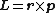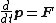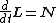# angular momentum

(redirected from Canonical angular momentum)
Also found in: Dictionary, Thesaurus, Medical.

## momentum

momentum (mōmĕnˈtəm), in mechanics, the quantity of motion of a body, specifically the product of the mass of the body and its velocity. Momentum is a vector quantity; i.e., it has both a magnitude and a direction, the direction being the same as that of the velocity vector. When an external force acts upon a body or a system of bodies in motion, it causes a change in the momentum of the body. The impulse of a force acting on a body is the product of the force and the duration of time in which it acts and is equal to the change in momentum of the body. When no external force acts upon a body in motion or a system of bodies there is no change in the total momentum even though, as in the case of a system of bodies, there may be an internal disturbance of the system resulting in changes in the momenta of individual bodies. This conclusion is commonly known as the principle of the conservation of momentum (see conservation laws, in physics). The momentum of a body should not be confused with its kinetic energy. The distinction between them can be seen in the action of a pile driver. The distance to which the pile is driven depends upon its kinetic energy; the length of time required for the action to cease, upon its momentum. In addition to the momentum a body has because of its linear motion, the body may also have angular momentum because of rotation. The angular momentum of a particle rotating about a point is equal to the product of the mass of the particle, its angular velocity, and the square of its distance from the axis of rotation. More simply, the angular momentum is the product of the instantaneous linear momentum and the distance. Angular momentum is a vector quantity directed perpendicular to the plane of motion.

## Angular momentum

In classical physics, the moment of linear momentum about an axis. A point particle with mass m and velocity v has linear momentum p = m v . Let r be an instantaneous position vector that locates the particle from an origin on a specified axis. The angular momentum L can be written as the vector cross-product in Eq. (1). (1)See Momentum

The time rate of change of the angular momentum is equal to the torque N . A rigid body satisfies two independent equations of motion (the dynamical equations) given by

(2)(3)Eqs. (2) and (3), where d/dt denotes the rate of change, the derivative with respect to time t. Only Eq. (2) is required for a point particle. Equation (2) indicates that a rigid body acts as a point particle located at its center of mass. The motion of the center of mass depends upon the net force F , which is the vector sum of all applied forces. Equation (3) gives the angular motion about the center of mass. The case of statics occurs when the net force and net torque both vanish. See Kinetics (classical mechanics), Statics, Torque

A symmetry is a transformation that leaves a physical system unchanged. A physical quantity is called invariant under a transformation if it remains the same after being transformed. For example, the solutions to Eqs. (2) and (3) are invariant under change of the coordinate origin or orientation of the i , j , and k axes. The freedom to choose any orientation of coordinate axes is called rotational invariance, because one choice of axes can be rotated into another. In physics, the rotational invariance follows from the isotropy and homogeneity of space that has been experimentally established to high accuracy.

The study of symmetry shows that one of the deepest relations in physics is that between dynamics and conservation. A physical quantity is conserved if it is constant in time, although it may vary in space. Noether's theorem states that if a physical system is invariant under a continuous symmetry, a conservation law exists, provided that the observable in question decreases rapidly enough at infinity. Thus, when the force is zero everywhere (the system is invariant under translation in space), the linear momentum is conserved. If the torque is zero everywhere (the system is invariant under rotation), the angular momentum is conserved. If the system is invariant under translations in time, the total energy is conserved. See Conservation laws (physics), Conservation of energy, Conservation of momentum

Quantum mechanics has a richer and more complicated structure than classical physics. Because of this, the relationship between symmetry and conservation is even more useful.

McGraw-Hill Concise Encyclopedia of Physics. © 2002 by The McGraw-Hill Companies, Inc.

## angular momentum

Symbol: L . A property of any rotating or revolving system whose value depends on the distribution of mass and velocity about the axis of rotation or revolution. It is a vector directed along the axis and for a body orbiting about a point it is given by the vector product of the body's linear momentum and position vector r , i.e. it is the product m (v × r ). It is also expressed as the product of the moment of inertia (I) and angular velocity (ω) of the body.

In a closed system, such as the Solar System or an isolated star, there is always conservation of momentum.

Collins Dictionary of Astronomy © Market House Books Ltd, 2006
The following article is from The Great Soviet Encyclopedia (1979). It might be outdated or ideologically biased.

## Angular Momentum

(or moment of momentum), one of the measures of the mechanical motion of a mass point or of a system. The angular momentum plays a particularly important role in the study of rotational motion. Just as with the moment of a force, a distinction is made between the angular momentum about a center (a point) and about an axis.

All the formulas used in calculating the moment of a force are valid for calculating the angular momentum k of a mass point about a center O or an axis z if in the formulas the vector F is replaced by the linear momentum m v. Thus, ko = [r ⋅ m v], where r is the radius vector of the moving point drawn from the center O and kz is equal to the projection of the ko vector onto a z-axis that passes through the point O. The angular momentum of a point varies under the action of the moment mo(F) of the applied force, and this variation is determined by the theorem on variation of angular momentum, which is expressed by the equation dko/dt = mo(F). When mo(F) = 0, as, for example, is the case for central forces, the motion of a point obeys the law of areas. This result is important in various fields, such as the theory of the motion of spacecraft and artificial earth satellites and celestial mechanics.

The principal angular momentum of a mechanical system about the center O or the z-axis is equal to the geometric or algebraic sum of the angular momenta of all the points of the system, respectively, about the same center or axis, that is, Ko = Σ oi and Kz = Σkzi. The vector Ko can be determined from its projections Kx, Ky, and Kz on the coordinate axes. For a body rotating about a fixed z-axis with an angular velocity ω, Kx = –Ixzω, Ky = – Iyzω, and Kz = Izω, where Iz is the moment of inertia about the z-axis, and Ixz and Iyz are the products of inertia. If the z-axis is the principal axis of inertia for the origin O, then Ko = Izω.

The principal angular momentum of a system varies only under the action of external forces, and this variation depends on the principal moment Meo of the external forces. This dependence is determined by the theorem on the variation of the principal angular momentum of a system, which is expressed by the equation dKo/dt = Meo The moments Kz and Mez about the z-axis are related by a similar equation. If Meo = 0 or Mez = 0, then Ko or K z, respectively, will be constant, that is, the law of conservation of angular momentum will hold. Thus, internal forces cannot change the angular momentum of a system, but the angular momenta of separate parts of the system or the angular velocity of the system may change under the influence of these forces. For example, for a figure skater rotating about a vertical z-axis, the quantity Kz= Izω will be constant, because Mez = 0 in practice. But by changing the value of his moment of inertia Iz by moving his arms or legs, the skater can vary his angular velocity ω. The appearance of a reactive moment in an engine with a rotating shaft (rotor) is another example of the validity of the law of conservation of angular momentum. The concept of angular momentum is used extensively in rigid-body dynamics, especially in the theory of gyroscopes.

Angular momentum has the dimensions L2MT−1; the units of measurement are kg-m2/sec or g⋅cm2/sec. Electromagnetic, gravitational, and other physical fields also have angular momenta. Most elementary particles have an intrinsic angular momentum—also called spin. The concept of angular momentum is of great importance in quantum mechanics.

S. M. TARG

The Great Soviet Encyclopedia, 3rd Edition (1970-1979). © 2010 The Gale Group, Inc. All rights reserved.

## angular momentum

[′aŋ·gyə·lər mə′ment·əm]
(mechanics)
The cross product of a vector from a specified reference point to a particle, with the particle's linear momentum. Also known as moment of momentum.
For a system of particles, the vector sum of the angular momenta (first definition) of the particles.
McGraw-Hill Dictionary of Scientific & Technical Terms, 6E, Copyright © 2003 by The McGraw-Hill Companies, Inc.
Site: Follow: Share:
Open / Close